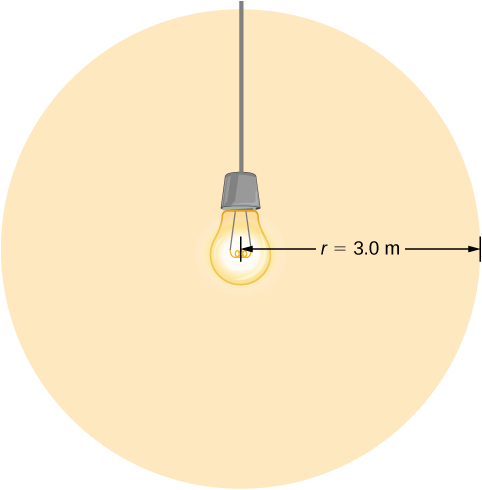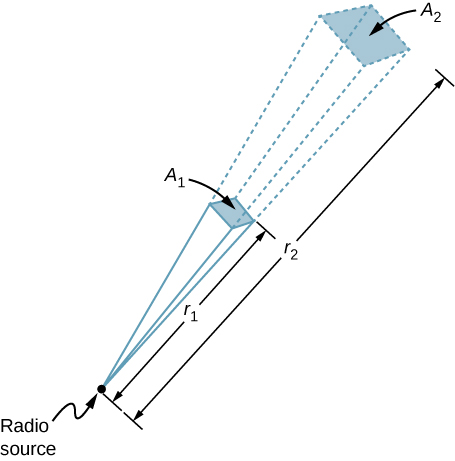# 16.3 Energy carried by electromagnetic waves  (Page 3/5)

 Page 3 / 5

## A laser beam

The beam from a small laboratory laser typically has an intensity of about $1.0\phantom{\rule{0.2em}{0ex}}×\phantom{\rule{0.2em}{0ex}}{10}^{-3}{\phantom{\rule{0.2em}{0ex}}\text{W/m}}^{2}$ . Assuming that the beam is composed of plane waves, calculate the amplitudes of the electric and magnetic fields in the beam.

## Strategy

Use the equation expressing intensity in terms of electric field to calculate the electric field from the intensity.

## Solution

From [link] , the intensity of the laser beam is

$I=\frac{1}{2}c{\epsilon }_{0}{E}_{0}^{2}.$

The amplitude of the electric field is therefore

${E}_{0}=\sqrt{\frac{2}{c{\epsilon }_{0}}I}=\sqrt{\frac{2}{\left(3.00\phantom{\rule{0.2em}{0ex}}×\phantom{\rule{0.2em}{0ex}}{10}^{8}\phantom{\rule{0.2em}{0ex}}\text{m/s}\right)\left(8.85\phantom{\rule{0.2em}{0ex}}×\phantom{\rule{0.2em}{0ex}}{10}^{-12}\phantom{\rule{0.2em}{0ex}}\text{F/m}\right)}\left(1.0\phantom{\rule{0.2em}{0ex}}×\phantom{\rule{0.2em}{0ex}}{10}^{-3}{\phantom{\rule{0.2em}{0ex}}\text{W/m}}^{2}\right)}=0.87\phantom{\rule{0.2em}{0ex}}\text{V/m}.$

The amplitude of the magnetic field can be obtained from [link] :

${B}_{0}=\frac{{E}_{0}}{c}=2.9\phantom{\rule{0.2em}{0ex}}×\phantom{\rule{0.2em}{0ex}}{10}^{-9}\phantom{\rule{0.2em}{0ex}}\text{T}.$

## Light bulb fields

A light bulb emits 5.00 W of power as visible light. What are the average electric and magnetic fields from the light at a distance of 3.0 m?

## Strategy

Assume the bulb’s power output P is distributed uniformly over a sphere of radius 3.0 m to calculate the intensity, and from it, the electric field.## Solution

The power radiated as visible light is then

$\begin{array}{ccc}\hfill I& =\hfill & \frac{P}{4\pi {r}^{2}}=\frac{c{\epsilon }_{0}{E}_{0}^{2}}{2},\hfill \\ \hfill {E}_{0}& =\hfill & \sqrt{2\frac{P}{4\pi {r}^{2}c{\epsilon }_{0}}}=\sqrt{2\frac{5.00\phantom{\rule{0.2em}{0ex}}\text{W}}{4\pi {\left(3.0\phantom{\rule{0.2em}{0ex}}\text{m}\right)}^{2}\left(3.00\phantom{\rule{0.2em}{0ex}}×\phantom{\rule{0.2em}{0ex}}{10}^{8}\phantom{\rule{0.2em}{0ex}}\text{m/s}\right)\left(8.85\phantom{\rule{0.2em}{0ex}}×\phantom{\rule{0.2em}{0ex}}{10}^{-12}{\phantom{\rule{0.2em}{0ex}}\text{C}}^{2}\text{/N}·{\text{m}}^{2}\right)}}=5.77\phantom{\rule{0.2em}{0ex}}\text{N/C,}\hfill \\ \hfill {B}_{0}& =\hfill & {E}_{0}\text{/}c=1.92\phantom{\rule{0.2em}{0ex}}×\phantom{\rule{0.2em}{0ex}}{10}^{-8}\phantom{\rule{0.2em}{0ex}}\text{T}.\hfill \end{array}$

## Significance

The intensity I falls off as the distance squared if the radiation is dispersed uniformly in all directions.

A 60-kW radio transmitter on Earth sends its signal to a satellite 100 km away ( [link] ). At what distance in the same direction would the signal have the same maximum field strength if the transmitter’s output power were increased to 90 kW?In three dimensions, a signal spreads over a solid angle as it travels outward from its source.

## Strategy

The area over which the power in a particular direction is dispersed increases as distance squared, as illustrated in the figure. Change the power output P by a factor of (90 kW/60 kW) and change the area by the same factor to keep $I=\frac{P}{A}=\frac{c{\epsilon }_{0}{E}_{0}^{2}}{2}$ the same. Then use the proportion of area A in the diagram to distance squared to find the distance that produces the calculated change in area.

## Solution

Using the proportionality of the areas to the squares of the distances, and solving, we obtain from the diagram

$\begin{array}{ccc}\hfill \frac{{r}_{2}^{2}}{{r}_{1}^{2}}& =\hfill & \frac{{A}_{2}}{{A}_{1}}=\frac{90\phantom{\rule{0.2em}{0ex}}\text{W}}{60\phantom{\rule{0.2em}{0ex}}\text{W}},\hfill \\ \hfill {r}_{2}& =\hfill & \sqrt{\frac{90}{60}}\left(100\phantom{\rule{0.2em}{0ex}}\text{km}\right)=122\phantom{\rule{0.2em}{0ex}}\text{km}.\hfill \end{array}$

## Significance

The range of a radio signal is the maximum distance between the transmitter and receiver that allows for normal operation. In the absence of complications such as reflections from obstacles, the intensity follows an inverse square law, and doubling the range would require multiplying the power by four.

## Summary

• The energy carried by any wave is proportional to its amplitude squared. For electromagnetic waves, this means intensity can be expressed as
$I=\frac{c{\epsilon }_{0}{E}_{0}^{2}}{2}$

where I is the average intensity in ${\text{W/m}}^{2}$ and ${E}_{0}$ is the maximum electric field strength of a continuous sinusoidal wave. This can also be expressed in terms of the maximum magnetic field strength ${B}_{0}$ as

$I=\frac{c{B}_{0}^{2}}{2{\mu }_{0}}$

and in terms of both electric and magnetic fields as

$I=\frac{{E}_{0}{B}_{0}}{2{\mu }_{0}}.$

The three expressions for ${I}_{\text{avg}}$ are all equivalent.

## Conceptual questions

When you stand outdoors in the sunlight, why can you feel the energy that the sunlight carries, but not the momentum it carries?

The amount of energy (about ${100\phantom{\rule{0.2em}{0ex}}\text{W/m}}^{2}$ ) is can quickly produce a considerable change in temperature, but the light pressure (about $3.00\phantom{\rule{0.2em}{0ex}}×\phantom{\rule{0.2em}{0ex}}{10}^{-7}{\text{N/m}}^{2}$ ) is much too small to notice.

what is principle of superposition
what are questions that are likely to come out during exam
what is electricity
watt is electricity.
electricity ka full definition with formula
Jyoti
If a point charge is released from rest in a uniform electric field will it follow a field line? Will it do so if the electric field is not uniform?
Maxwell's stress tensor is
Yes
doris
neither vector nor scalar
Anil
if 6.0×10^13 electrons are placed on a metal sphere of charge 9.0micro Coulombs, what is the net charge on the sphere
18.51micro Coulombs
ASHOK
Is it possible to find the magnetic field of a circular loop at the centre by using ampere's law?
Is it possible to find the magnetic field of a circular loop at it's centre?
yes
Brother
The density of a gas of relative molecular mass 28 at a certain temperature is 0.90 K kgmcube.The root mean square speed of the gas molecules at that temperature is 602ms.Assuming that the rate of diffusion of a gas in inversely proportional to the square root of its density,calculate the density of
A hot liquid at 80degree Celsius is added to 600g of the same liquid originally at 10 degree Celsius. when the mixture reaches 30 degree Celsius, what will be the total mass of the liquid?
Under which topic
doris
what is electrostatics
Study of charges which are at rest
himanshu
Explain Kinematics
Two equal positive charges are repelling each other. The force on the charge on the left is 3.0 Newtons. Using your notes on Coulomb's law, and the forces acting on each of the charges, what is the force on the charge on the right?
Using the same two positive charges, the left positive charge is increased so that its charge is 4 times LARGER than the charge on the right. Using your notes on Coulomb's law and changes to the charge, once the charge is increased, what is the new force of repulsion between the two positive charges?
Nya
A mass 'm' is attached to a spring oscillates every 5 second. If the mass is increased by a 5 kg, the period increases by 3 second. Find its initial mass 'm'
a hot water tank containing 50,000g of water is heated by an electric immersion heater rated at 3kilowatt,240volt, calculate the current
what is charge
product of current and time
JaffarBy Samuel MaddenBy OpenStaxBy Donyea SweetsBy Yasser IbrahimBy OpenStaxBy Mahee BooBy Richley CrapoBy Marion CabalfinBy OpenStaxBy Brooke Delaney